Ex 5.2

Chapter 5 Class 11 Complex Numbers
Serial order wise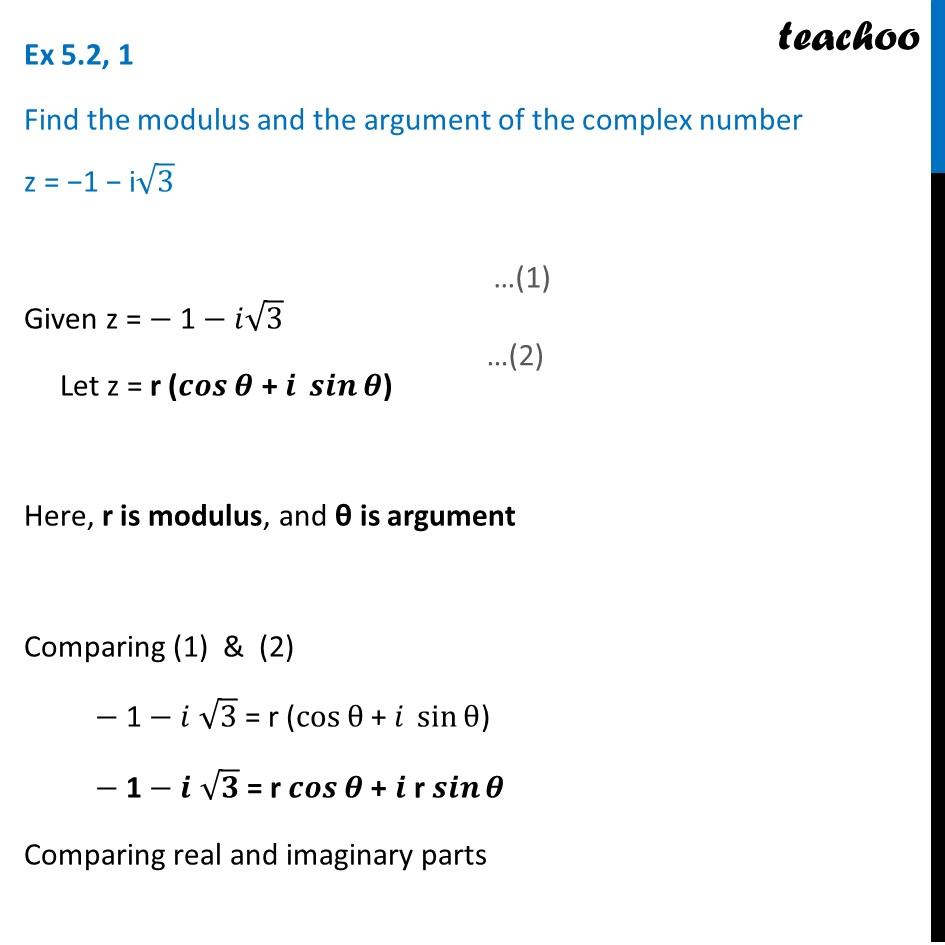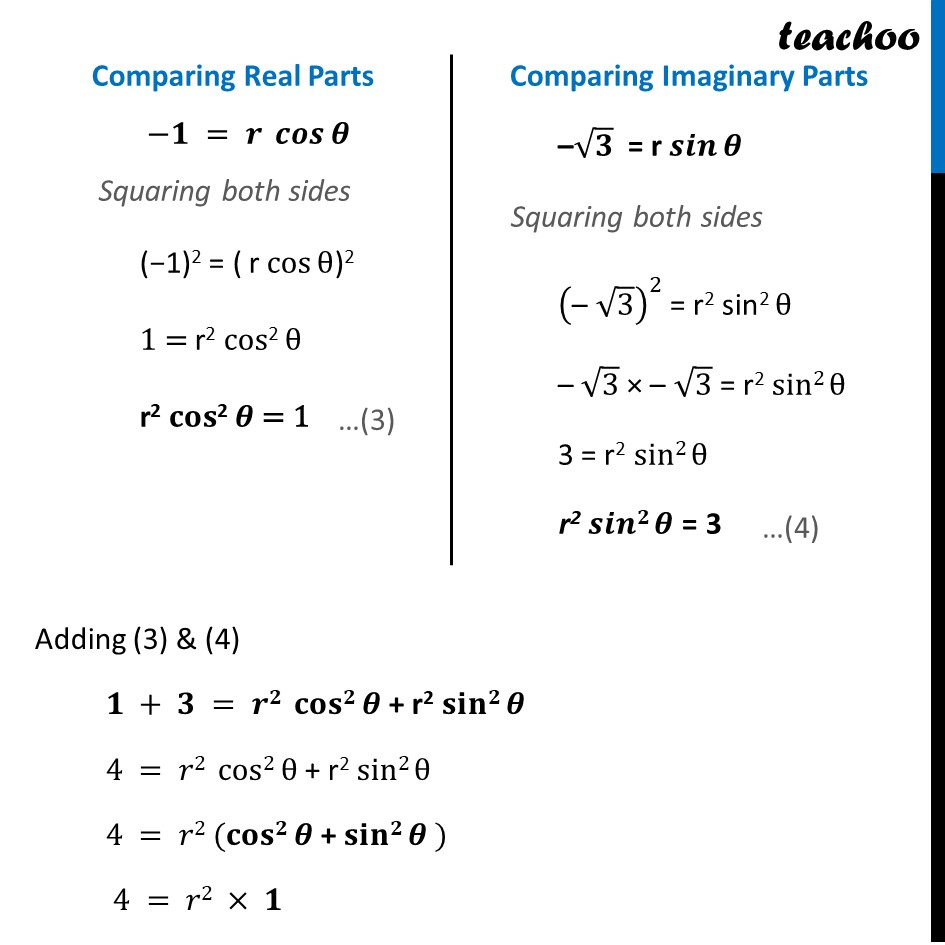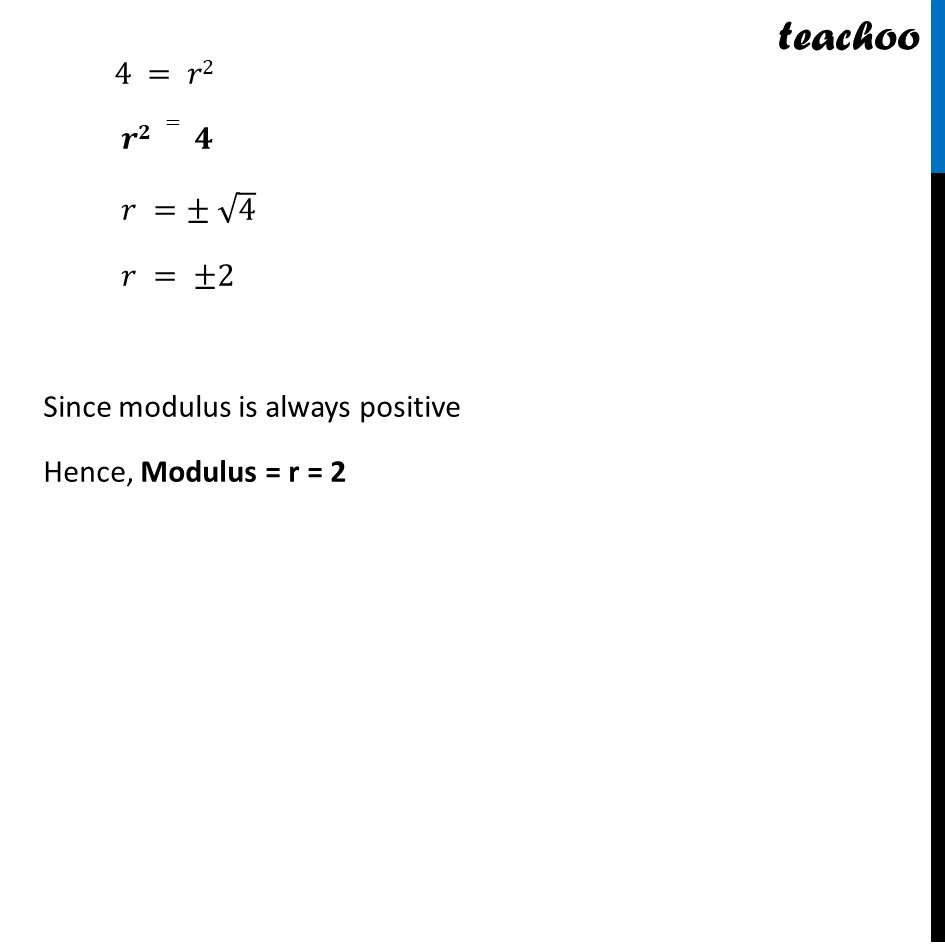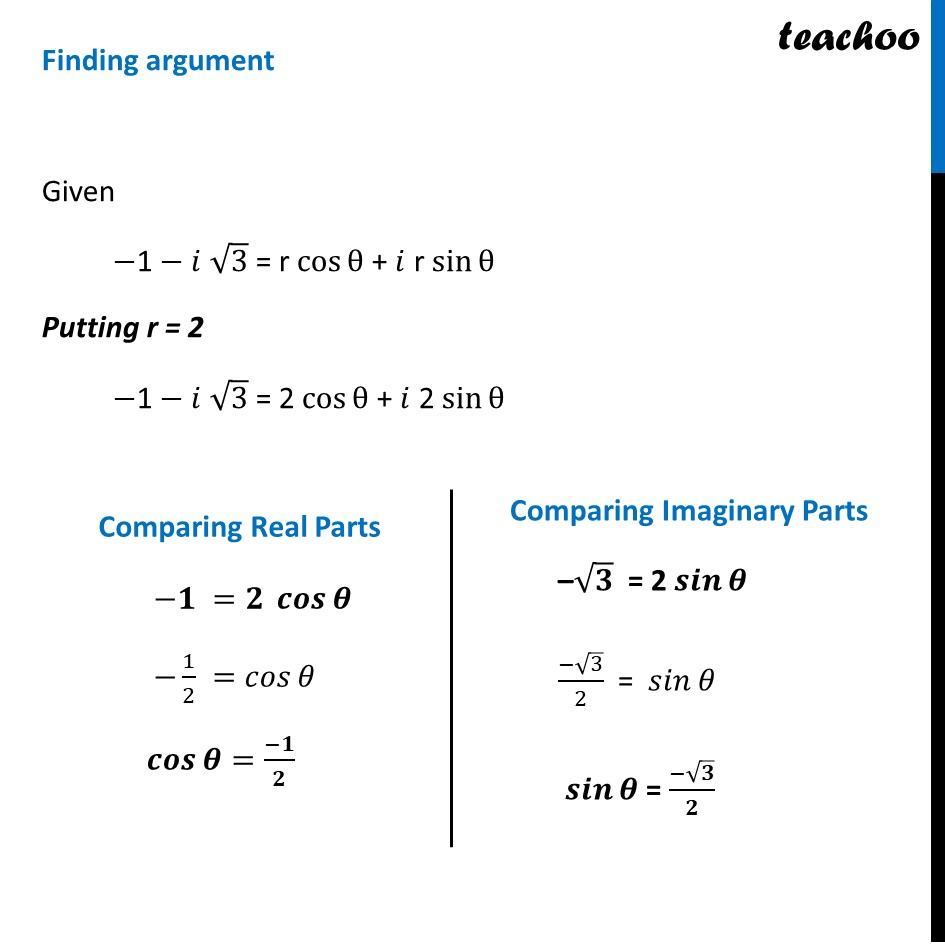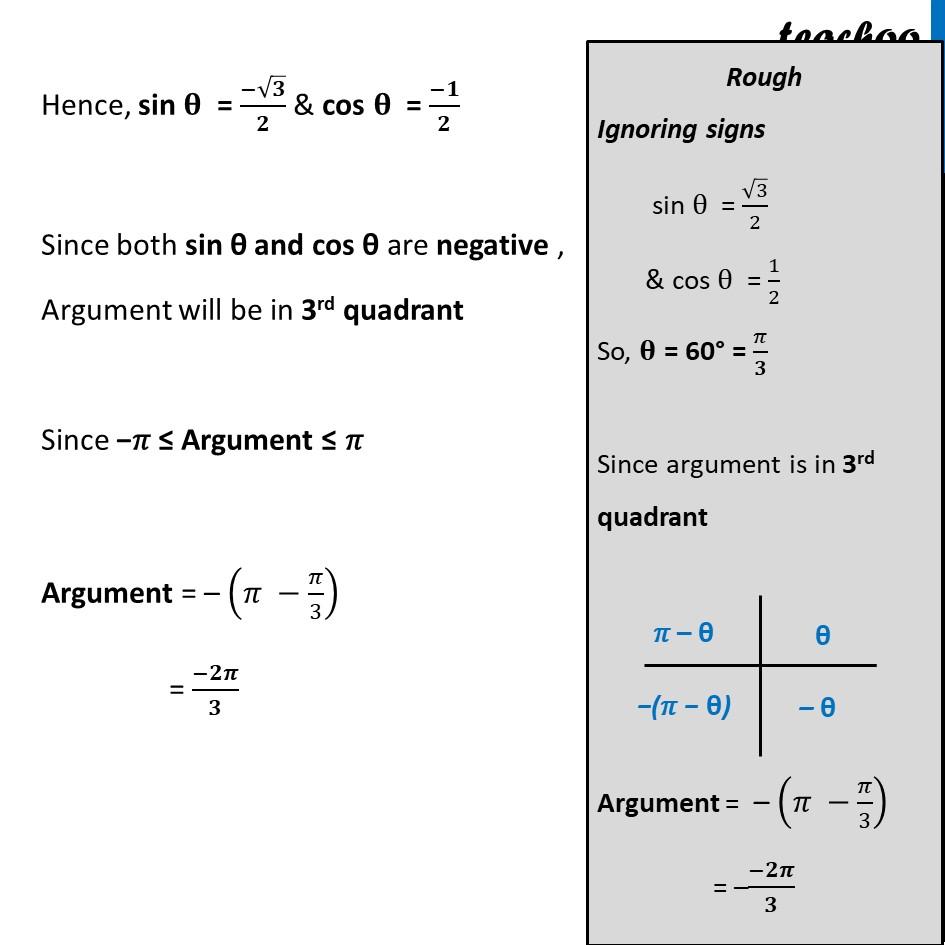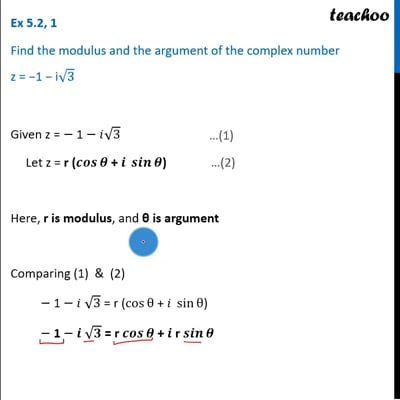This video is only available for Teachoo black users

Maths Crash Course - Live lectures + all videos + Real time Doubt solving!

### Transcript

Ex 5.2, 1 Find the modulus and the argument of the complex number z = −1 − i√3 Given z = − 1 − 𝑖√3 Let z = r (𝒄𝒐𝒔⁡𝜽 + 𝒊 𝒔𝒊𝒏⁡𝜽) Here, r is modulus, and θ is argument Comparing (1) & (2) − 1 − 𝑖 √3 = r (cos⁡θ + 𝑖 sin⁡θ) − 1 − 𝒊 √𝟑 = r〖 𝒄𝒐𝒔〗⁡𝜽 + 𝒊 r 𝒔𝒊𝒏⁡𝜽 Comparing real and imaginary parts Comparing Real Parts −𝟏 = 𝒓 𝒄𝒐𝒔⁡𝜽 Squaring both sides (−1)2 = ( r〖 cos〗⁡θ)2 1 = r2〖 cos"2" 〗⁡θ r2〖 𝐜𝐨𝐬"2" 〗⁡𝜽 = 1 Comparing Imaginary Parts –√𝟑 = r〖 𝒔𝒊𝒏〗⁡𝜽 Squaring both sides ("– " √3)^2 = r2 sin2 θ – √3 × – √3 = r2 sin2⁡θ 3 = r2 sin2⁡θ r2 𝒔𝒊𝒏𝟐⁡𝜽 = 3 Adding (3) & (4) 𝟏 + 𝟑 = 𝒓𝟐 𝐜𝐨𝐬𝟐⁡𝜽 + r2 𝐬𝐢𝐧𝟐⁡𝜽 4 = 𝑟2 cos2⁡θ + r2 sin2⁡θ 4 = 𝑟2 (𝐜𝐨𝐬𝟐⁡𝜽 + 𝐬𝐢𝐧𝟐⁡𝜽 ) 4 = 𝑟2 × 𝟏 4 = 𝑟2 𝒓𝟐 = 𝟒 𝑟 =± √4 𝑟 = ±2 Since modulus is always positive Hence, Modulus = r = 2 Finding argument Given −1 − 𝑖 √3 = r〖 cos〗⁡θ + 𝑖 r sin⁡θ Putting r = 2 −1 − 𝑖 √3 = 2〖 cos〗⁡θ + 𝑖 2 sin⁡θ Comparing Real Parts −𝟏 =𝟐 𝒄𝒐𝒔⁡𝜽 −1/2 =𝑐𝑜𝑠⁡𝜃 𝒄𝒐𝒔⁡𝜽=(−𝟏)/𝟐 Comparing Imaginary Parts –√𝟑 = 2〖 𝒔𝒊𝒏〗⁡𝜽 (−√3)/2 = 〖 𝑠𝑖𝑛〗⁡𝜃 〖 𝒔𝒊𝒏〗⁡𝜽 = (−√𝟑)/𝟐 Hence, sin 𝛉 = (−√𝟑)/𝟐 & cos 𝛉 = (−𝟏)/𝟐 Since both sin θ and cos θ are negative , Argument will be in 3rd quadrant Since −𝜋 ≤ Argument ≤ 𝜋 Argument = – (𝜋 −𝜋/3) = (−𝟐𝝅)/𝟑